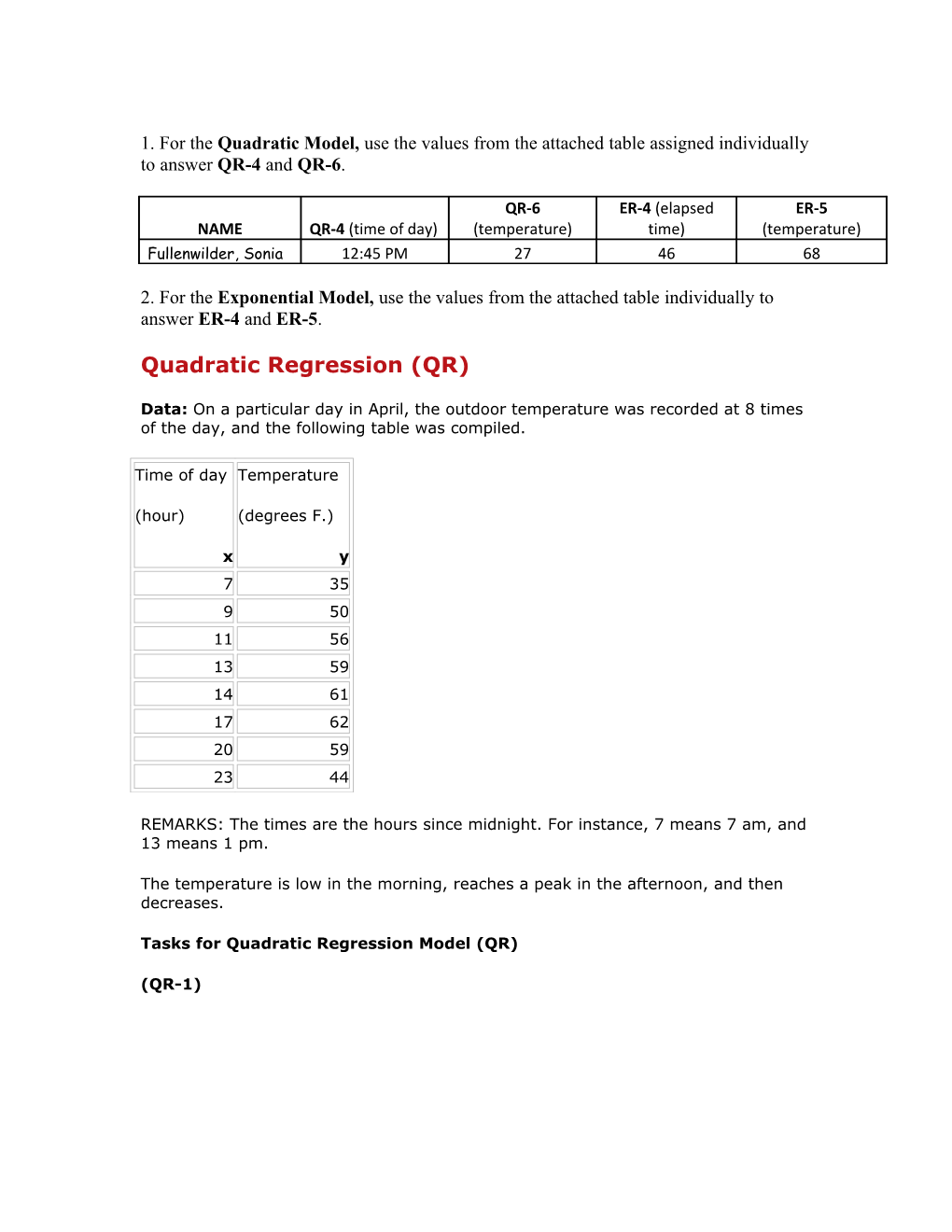1. For theQuadratic Model,use the values from the attached table assigned individually to answer QR-4 and QR-6.

NAME / QR-4 (time of day) / QR-6 (temperature) / ER-4 (elapsed time) / ER-5 (temperature)
Fullenwilder, Sonia / 12:45 PM / 27 / 46 / 68

2. For theExponential Model,use the valuesfrom the attached tableindividually to answer ER-4 and ER-5.

Data:On a particular day in April, the outdoor temperature was recorded at 8 times of the day, and the following table was compiled.

Time of day
(hour)
x / Temperature
(degrees F.)
y
7 / 35
9 / 50
11 / 56
13 / 59
14 / 61
17 / 62
20 / 59
23 / 44

REMARKS: The times are the hours since midnight. For instance, 7 means 7 am, and 13 means 1 pm.

The temperature is low in the morning, reaches a peak in the afternoon, and then decreases.

(QR-1)We can note that the trend is definitely non-linear.

(QR-2)Quadratic polynomial of best fit is y = -0.3476x2 + 10.948x - 23.078

where x = Time of day in hours and y = Temperature in degrees

(QR-3)

r2 = 0.9699

The closerr2is to 1, the better the fit. For this model, value of r2 is close to 1 so the model fits the data well.

(QR-4)

Time of day = 12:45 PM

Time of day in hours = 12.75 hours

Put x = 12.75 in y = -0.3476x2 + 10.948x - 23.078

y = -0.3476x2 + 10.948x - 23.078

y = -0.3476(12.75)2 + 10.948(12.75) - 23.078 ≈ 60°F

Estimated outdoor temperature at 12:45 PM is 60°F.

Time of day in hours = 12.75 hours

Estimated outdoor temperature = 60°F

(QR-5)

y = -0.3476x2 + 10.948x - 23.078

Temperature will be maximum at the vertex of the parabola.

Vertex will be atx = -b/(2a) = -10.948/(2*(-0.3476)) = 15.75 hours

Time of day at maximum temperature = 15.75 hours

Maximum temperature

= y(15.75)

= -0.3476(15.75)2 + 10.948(15.75) - 23.078

= 63.1°F

Maximum temperature is 63.1°F.

15.75 hours is the same as 3:45 PM

Maximum temperature = 63.1°F

Time of day = 3:45 PM

(QR-6)

I have to find time when temperature is 27ºF:

27 = -0.3476x2 + 10.948x - 23.078

0.3476x2 - 10.948x + 23.078 + 27 = 0

0.3476x2 - 10.948x + 50.078 = 0

Rounding to the nearest quarter hour, x = 5.50, 26.0

26 is the same as 2

Rounding to the nearest quarter hour, the temperature will be 27ºF at 5:30AM and 2 AM.

Targeted temperature = 27ºF

Time(s) of day = 2 AM, 5:30 AM

Exponential Regression (ER)

Data:A cup of hot coffee was placed in a room maintained at a constant temperature of 69 degrees.

The temperature of the coffee was recorded periodically, and the following table was compiled.

Table 1:

Time Elapsed
(minutes) / Coffee
Temperature
(degrees F.)
x / T
0 / 166.0
10 / 140.5
20 / 125.2
30 / 110.3
40 / 104.5
50 / 98.4
60 / 93.9

REMARKS: Common sense tells us that the coffee will be cooling off and its temperature will decrease and approach the ambient temperature of the room, 69 degrees.

So, the temperature difference between the coffee temperature and the room temperature will decrease to 0.

We will be fitting the data to an exponential curve of the formy=Ae-bx. Notice that asxgets large,ywill get closer and closer to 0, which is what the temperature difference will do.

So, we want to analyze the data wherex= time elapsed andy=T- 69, the temperature difference between the coffee temperature and the room temperature.

Table 2

Time Elapsed
(minutes) / Temperature
Difference
(degrees F.)
x / y
0 / 97.0
10 / 71.5
20 / 56.2
30 / 41.3
40 / 35.5
50 / 29.4
60 / 24.9

Tasks for Exponential Regression Model (ER)

(ER-1)We can note from the plot that the trend is definitely non-linear.

(ER-2)Exponential function of best fitis y = 89.976e-0.023x

where x = Time elapsed in minutes and y = Temperature difference in degrees F

Here A = 89.976 and b = 0.023

(ER-3)

Coefficient of determination r2 = 0.9848

The closerr2is to 1, the better the fit. For this model, value of r2 is close to 1 so the model fits the data well.

(ER-4)

Given elapsed time = 46 minutes

y(46) = 89.976e-0.023(46) ≈ 31.24

Estimated coffee temperature T = y+69 = 31.24 +69 = 100.24degrees F

Elapsed time = 46 minutes

Estimated coffee temperature T = 100.24 degrees F

(ER-5)

Given temperature T = 68 degree F

y=T– 69 = 68-69 = -1 degree F

Now solve the equation

89.976e-0.023x = -1

e-0.023x = -1/89.976

We can’t take natural log of negative numbers so there will be no solution.